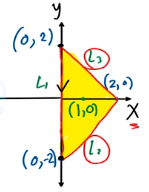Need Help?

Subscribe to Calculus C

###### \${selected_topic_name}
• Notes

Find the absolute maximum and minimum values of $f$ on the set $D .$
$f(x, y)=x^{2}+y^{2}-2 x, \quad D$ is the closed triangular region
with vertices $(2,0),(0,2),$ and $(0,-2)$

$f(x, y)=x^{2}+y^{2}-2 x$

$f_{x}(x, y)=2 x-2=0 \quad x=1$

$f_{y}\left(x, y\right)=2 y=0 \quad y=0$

$(1,0)$ Critical point

$f(x, y)=1+(-2)=-1$ Min

$L_{1}\quad x=0$

$f(0, y)=y^{2}$

$y=[-2,2]$

$(0, \pm 2)$

$f(0, \pm 2)=y^{2}=( \pm 2)^{2}=4$ Max

Max $\Rightarrow 4$ @ $(0,\pm2)$
Min $\Rightarrow-1$ @ $(1,0)$